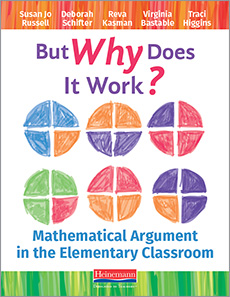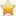# But Why Does It Work?

## Mathematical Argument in the Elementary Classroom

Getting students to explain and support their mathematical thinking is rarely easy. But Why Does It Work? explores a teaching model that takes the mystery out of this challenge! You'll learn how focusing on mathematical argument develops your students' ability to justify their thinking and engage with the reasoning of others.Special Offer: Save 30% off our list price automatically when you buy 15 or more.

### BOOK WITH ONLINE

In Stock

List Price: \$31.25

Web/School Price: \$25.00

ALSO AVAILABLE AS:
eBook
eBook + Print Bundle

### Full Description

If you ask students, "Why does that work?" do they know what you're asking and do you know what to listen for in their responses? Do you have images of what mathematical argument looks like in the elementary grades and how to help students learn to engage in this important practice? Do you have so much content to cover that finding time for this kind of work is difficult?

But Why Does It Work? offers a simple, efficient teaching model focused on mathematical argument for developing the ability of students to justify their thinking and engage with the reasoning of others. Designed for individuals as well as study groups, this book includes access to classroom-ready instructional sequences, each built on a model supporting students in:

• noticing relationships across sets of problems, equations, or expressions
• articulating a claim about what they notice
• investigating their claim through representations such as manipulatives, diagrams, or story contexts
• using their representations to demonstrate why a claim must be true or not
• extending their thinking from one operation to another.

Establishing a classroom culture where students gain confidence in their own mathematical voice and learn to value the contributions of their peers is a critical part of this work. The authors tell us, “If the idea underlying a student’s reasoning is not made explicit, the opportunity for all students to engage in such thinking is lost.” As students become a true community of mathematicians, they heighten each other’s understanding by investigating questions, conjectures, and examples together.

Enhanced with extensive video showing the instructional sequences in action—along with guiding focus questions and math investigations—But Why Does It Work? is a flexible approach that will help students confidently articulate and defend their reasoning, and share their deep thinking with others.

(click any section below to continue reading)

### Contents

Introduction

• Content of the Book
• Classroom Examples: Text and Video
• The Lesson Sequences on Mathematical Argument

Chapter 1 Mathematical Argument in the Elementary Grades: What and Why?

• The What: Representation-Based Argument
• Examples of Mathematical Argument
• Why Linger on Mathematical Argument?

Chapter 2 Elementary Students as Mathematicians

• The Mathematician's Process
• Facilitating a Community of Student Mathematicians
• Why Should Students Act Like Mathematicians?

Chapter 3 The Teaching Model

• Introducing the Lesson Sequence
• Phase I: Noticing Regularity
• Phase II: Articulating a Claim
• Phase III: Investigating Through Representations
• Phase IV: Constructing Arguments
• Phase V: Comparing Operations
• What Students Gain

Chapter 4 Using the Lesson Sequences: What the Teacher Does

• Phase I: Noticing Regularity
• Phase II: Articulating a Claim
• Phase III: Investigating Through Representations
• Phase IV: Constructing Arguments
• Phase V: Comparing Operations

Chapter 5 Mathematical Argument in the Elementary Classroom: Impact on Students and Teachers

• Mathematics Content and Practices
• Applying New Understanding in Students' Regular Math Work
• Participation and Confidence Among the Range of Learners
• Mathematical Argument: An Opportunity for Teacher Learning About Fundamental Mathematics

Appendix A Same Sum, Same Difference (Whole Numbers)

• Goals for Students
• Sequence Overview

Appendix B Changing a Number in Addition of Multiplication (Whole Numbers)

• Goals for Students
• Sequence Overview

Online Lesson Sequences

• Sequence 1: Core Ideas of Addition and Subtraction (Whole Numbers)
• Sequence 2: Changing a Number in Addition or Subtraction (Whole Numbers)
• Sequence 3: Same Sum, Same Difference (Whole Numbers)
• Sequence 4: Core Ideas of Multiplication and Division (Whole Numbers)
• Sequence 5: Changing a Number in Addition or Multiplication (Whole Numbers)
• Sequence 6: Factors, Products, and Fractions (Whole Numbers and Fractions)
• Sequence 7: Same Product, Same Quotient (Whole Numbers)
• Sequence 8: Multiplication, Division, and Powers of Ten (Wholes Numbers and Decimals)

### In Depth

Chapter 1, “Mathematical Argument in the Elementary Grades: What and Why?,” introduces the major content of the book—the focus on mathematical argument, why that’s important, and the choice of operations as a context for work on mathematical argument for elementary students. The chapter introduces the concept of “productive lingering” on important mathematics. Chapter 2, “Elementary Students as Mathematicians,” describes how students engage in “new to them” mathematics and how their work parallels the process by which professional mathematicians create mathematical ideas. Chapter 3, “The Teaching Model,” presents the teaching model for engaging students in mathematical argument, describes the five phases in detail, and presents classroom examples to illustrate how each phase engages students in important mathematical ideas and practices. Chapter 4, “Using the Lesson Sequences: What the Teacher Does,” details where the teacher focuses her attention, the decisions she makes in response to students’ thinking, and the actions she takes as the class goes through the fives phases of the teaching model. Chapter 5, “Mathematical Argument in the Elementary Classroom: Impact on Students and Teachers,” summarizes evidence from student assessments and classroom observations about what students in our collaborating classrooms learned about mathematics content and practices, what was gained in participation and confidence, and how students applied their learning about mathematical argument in their regular math classes.

Chapters 1 through 4 also include video- and text-based examples drawn from the classrooms of our collaborating teachers; mathematical investigations for you to develop your own mathematical thinking and to become familiar with the mathematics content students in the examples are working on; and sets of focus questions about selected examples and about each chapter as a whole.

— Introduction, xii-xiii